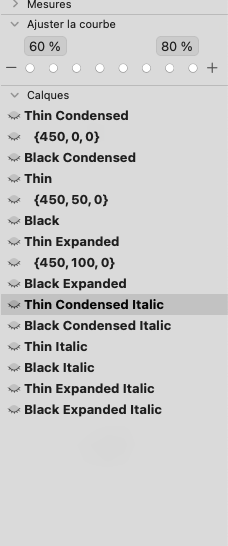# Change several values of a several brace layer

Hi, I would know if someone can provide a script to change several special layer with several axis for all the font.
E.g
From
{400, 0, 0} to {400, 50, 0}
{400, 50, 0} to {400, 100, 0}

Yes is for update the correct width valueThank you!

To change the axis coordinates for all intermediate layers that have a specific location:

``````current_axis_1 = 400
current_axis_2 = 0
curren_axis_3 = 0

desired_axis_1 = 400
desired_axis_2 = 0
desired_axis_3 = 50

desired_coordinates = [desired_axis_1, desired_axis_2]

for glyph in Font.glyphs:
for layer in glyph.layers:
if not layer.isSpecialLayer:
continue
if not layer.attributes["coordinates"]:
continue
layer_coordinates = [layer.attributes["coordinates"][axis.axisId] for axis in Font.axes]
if layer_coordinates == [current_axis_1, current_axis_2, current_axis_3]:
for index, axis in enumerate(Font.axes):
layer.attributes["coordinates"][axis.axisId] = desired_coordinates[index]
``````

If you want to change all width axis values for all intermediate layers in your selected glyphs, run this (select the glyphs for which you want to update the values, then run the script):

``````for selection in Font.selectedLayers:
for layer in selection.parent.layers:
if not layer.isSpecialLayer:
continue
if not layer.attributes["coordinates"]:
continue
layer_coordinates = [layer.attributes["coordinates"][axis.axisId] for axis in Font.axes]
if layer_coordinates == 0:
layer.attributes[Font.axes.axisId] = 50
if layer_coordinates == 50:
layer.attributes[Font.axes.axisId] = 100
``````

You should install Rainers collection of scripts. You can do that from the Plugin Manager.
Then you can search for say, ‘brace’ in the Glyphs help menu:

And you will find Rainer’s script

1 Like

Thank you.
I will try both solution

Rainer script works for only the first axe value

You select the axis you want the script to work on in the field after ‘for axis at index’. Remember that your first axis is index 0.

Hou right, I didn’t notice this index. Thank you !

1 Like

Hi, thank you fo theses script.
However, I try them and the first one doesn’t works even if I corrected
if layer_coordinates == [axis_1, axis_2]:
with
if layer_coordinates == [desired_axis_1, desired_axis_2]:

No console error but nor result.

Same for the second one

What version of Glyphs are you using? Are the values you provided exactly the ones in your font?

I have 3.1.2 (3151)
Yes I provide exactly the value of my font like that:

``````current_axis_1 = 450
current_axis_2 = 0
curren_axis_3 = 0

desired_axis_1 = 450
desired_axis_2 = 51
desired_axis_3 = 0

desired_coordinates = [desired_axis_1, desired_axis_2, desired_axis_3]

for glyph in Font.glyphs:
for layer in glyph.layers:
if not layer.isSpecialLayer:
continue
if not layer.attributes["coordinates"]:
continue
layer_coordinates = [layer.attributes["coordinates"][axis.axisId] for axis in Font.axes]
if layer_coordinates == [desired_axis_1, desired_axis_2, desired_axis_3]:
for index, axis in enumerate(Font.axes):
layer.attributes["coordinates"][axis.axisId] = desired_coordinates[index]`````````

Can you show a screenshot of your brace layer with its coordinates?

What happens if you open your brace layer and run

``````print(Layer.attributes)
``````

with print:
{
coordinates = {
a01 = 450;
a02 = 0;
a03 = 0;
};
}Why did you change the line `if layer_coordinates = [current_axis_1, current_axis_2, current_axis_3]`?

Leave that line as it is. I fixed my initial code sample.